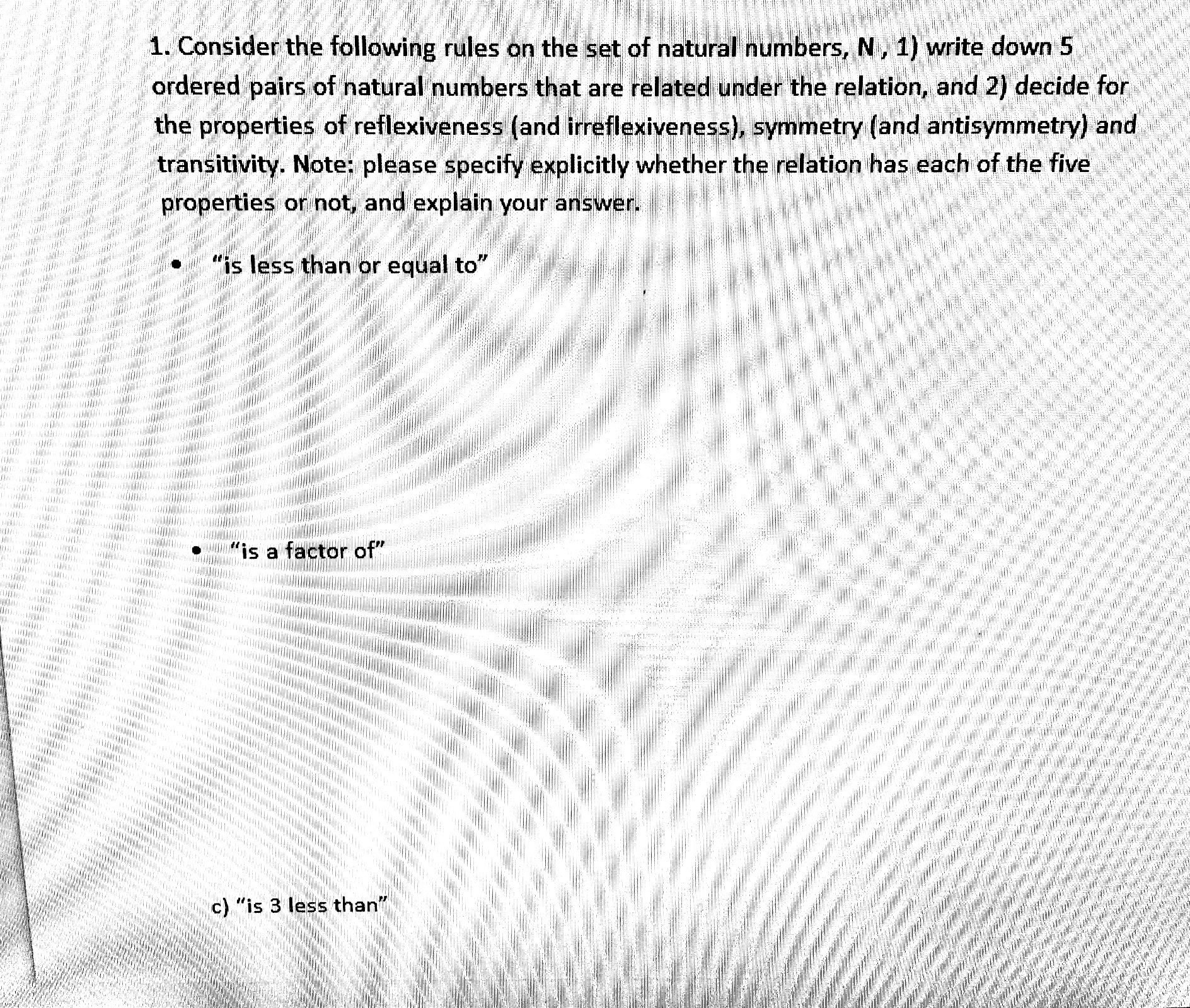# 1. Consider the following rules on the set of natural numbers, N, 1) write down 5ordered pairs of natural numbers that are related under the relation, and 2) decide forthe properties of reflexiveness (and irreflexiveness), symmetry (and antisymmetry) andtransitivity. Note: please specify explicitly whether the relation has each of the fiveproperties or not, and explain your answer."is less than or equal to"a factor of"c) "is 3 less than"

Question
61 viewshelp_outlineImage Transcriptionclose1. Consider the following rules on the set of natural numbers, N, 1) write down 5 ordered pairs of natural numbers that are related under the relation, and 2) decide for the properties of reflexiveness (and irreflexiveness), symmetry (and antisymmetry) and transitivity. Note: please specify explicitly whether the relation has each of the five properties or not, and explain your answer. "is less than or equal to" a factor of" c) "is 3 less than" fullscreen
check_circle

Step 1

The 5 order pair of the natural number that is satisfy the relation “less than or equal to” as follows,

That means, if a and b are the natural number then it satisfy (a b)

So, (1, 1), (1, 2), (2, 2), (2, 3), (1, 3)

When a, b and c belongs to the natural number or real number then,

If (a, a) or (b, b) or (c, c) ϵ N, then it reflexive.

If (a, b) ϵ N and also (b, a) ϵ N then it symmetry.

And, (a, b) ϵ N and (b, c) ϵ N then if (a, c) ϵ N, it is transitive.

Now, according to the above properties,

Since (1, 1) and (2, 2) are the reflexive. So the given relation is reflexive.

The above relation are not symmetric because a less than or equal to b but b not less than or equal to a.

Here, (1, 2) and (2, 3) and then also (1, 3) so the above relation is transitive.

Step 2

The 5 order pair of the natural number that is satisfy the relation “is a factor of” as follows,

That means, if a and b are the natural number then it satisfy (a factor of b)

So, (2, 4), (2, 6), (...

### Want to see the full answer?

See Solution

#### Want to see this answer and more?

Solutions are written by subject experts who are available 24/7. Questions are typically answered within 1 hour.*

See Solution
*Response times may vary by subject and question.
Tagged in

### Math